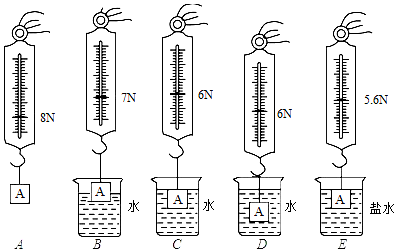（1）读数时应使圆柱体处于　 　状态。

（2）在C与E两图中，保持了排开液体的体积不变，研究浮力与　 　的关系；根据A与E两图所标的实验数据，可知物体浸没在盐水中所受的浮力为　 　N。

（3）小明对ABCD四个步骤进行了观察研究，发现浮力的大小有时与深度有关，有时与深度又无关对此正确的解释是浮力的大小随着排开水的体积的增大而　 　，当物体完全浸没在水中后排开水的体积相同，浮力的大小与深度　 　。

（4）在小明实验的基础上，根据有关实验数据，可以计算出盐水的密度为　 　kg/m3。

（2）在C与E两图中，保持了排开液体的体积不变，研究浮力与排开液体密度的关系；根据A与E两图所标的实验数据，可知物体浸没在盐水中所受的浮力F浮盐＝G﹣F＝8N﹣5.6N＝2.4N；

（3）由F浮＝ρ液gV排得，浮力的大小随着排开水的体积的增大而增大，当物体完全浸没在水中后排开水的体积相同，浮力的大小与深度无关；

（4）物体浸没在水中所受的浮力F浮水＝G﹣F＝8N﹣6N＝2N，

$$\frac{F_{\text {浮水 }}}{\rho_{\text {水 }} g}=\frac{F_{\text {浮盐 }}}{\rho_{\text {盐水 }} g}, \rho_{\text {盐水 }}=\frac{F_{\text {浮盐 }}}{F_{\text {浮水 }}} \cdot \rho_{\text {水 }}=\frac{2.4 \mathrm{~N}}{2 \mathrm{~N}} \times 10^3 \mathrm{~kg} / \mathrm{m}^3=1.2 \times 10^3 \mathrm{~kg} / \mathrm{m}^3 \text { 。 }$$

(2) 液体的密度; 2. 4;
(3) 增大; 无关;
(4) $1.2 \times 10^3$
①点击 收藏 此题， 扫码注册关注公众号接收信息推送（一月四份试卷,中1+高2+研1）
② 程序开发、服务器资源都需要大量的钱，如果你感觉本站好或者受到到帮助，欢迎赞助本站,赞助方式：微信/支付宝转账到 18155261033

 ①此题难易度如何 ②此题推荐度如何 确定